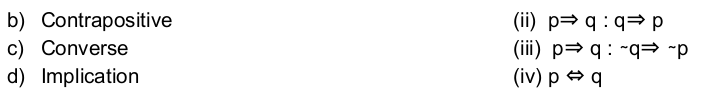# UGC-NET | UGC NET CS 2018 Dec – II | Question 19

• Last Updated : 02 Nov, 2021

Match the List 1 and List 2 and choose the correct answer from the code given below:(A) (a)-(i), (b)-(ii), (c)-(iii), (d)-(iv)
(B) (a)-(ii), (b)-(i),(c)-(iii), (d)-(iv)
(C) (a)-(iv), (b)-(iii), (c)-(ii), (d)-(i)
(D) (a)-(iii), (b)-(iv), (c)-(ii), (d)-(i)

Explanation: In particular, there are three related conditional statements that occur so often that they have special names.

• Implication : p → q
• Converse : The converse of the proposition p → q is q → p
• Contrapositive : The contrapositive of the proposition p → q is q’ → p’
• Inverse : The inverse of the proposition p → q is ‘p → q’

So, option (C) is correct.

Quiz of this Question

My Personal Notes arrow_drop_up